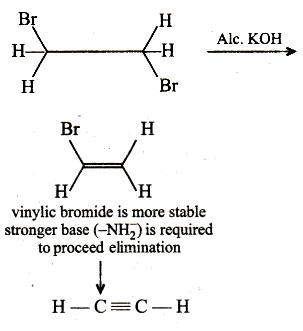Courses

# Sample JEE(MAIN) Chemistry Mock Test

## 30 Questions MCQ Test JEE Main Mock Test Series 2020 & Previous Year Papers | Sample JEE(MAIN) Chemistry Mock Test

Description
This mock test of Sample JEE(MAIN) Chemistry Mock Test for JEE helps you for every JEE entrance exam. This contains 30 Multiple Choice Questions for JEE Sample JEE(MAIN) Chemistry Mock Test (mcq) to study with solutions a complete question bank. The solved questions answers in this Sample JEE(MAIN) Chemistry Mock Test quiz give you a good mix of easy questions and tough questions. JEE students definitely take this Sample JEE(MAIN) Chemistry Mock Test exercise for a better result in the exam. You can find other Sample JEE(MAIN) Chemistry Mock Test extra questions, long questions & short questions for JEE on EduRev as well by searching above.
QUESTION: 1

### 1-Phenylethanol can be prepared by the reaction of benzaldehyde with

Solution: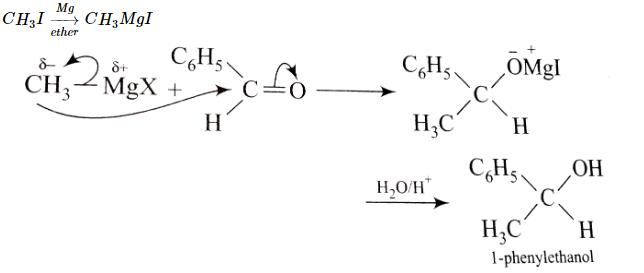QUESTION: 2

Solution:
QUESTION: 3

### Which is most ionic?

Solution:

For a specie to be ionic there must be -
large cation
small anion.
lesser the number of charge  which is there in MnO.

QUESTION: 4

Electrophiles are

Solution:

An electrophile is a reagent attracted to electrons. Electrophiles are positively charged or neutral species having vacant orbitals that are attracted to an electron rich centre. It participates in a chemical reaction by accepting an electron pair in order to bond to a nucleophile.

QUESTION: 5

The effect of atoms or groups loosing electrons towads a carbon atom is called

Solution: Polarity produced in a molecule due to transmission of unequal sharing/partial displacement of the bonding electron through a chain of atoms in molecules towards more electronegative atom, giving rise to a permanent dipole in a bond. It is present in a sigma bond, as opposed to the electromeric effect, which is present on a π-bond. it's called +I effect.
QUESTION: 6

Electronic configuration of deuterium atom is

Solution:

Deuterium is also known as "Heavy Hydrogen"
A Deuterium atom consists of a proton, a neutron and an electron.
It is just like the Hydrogen, but only with a. neutron.
So, electronic configuration is same as that of Hydrogen: 1s¹

QUESTION: 7

If principal quantum no. of atom is 3, then its azimuthal quantum number is

Solution: Principle quantum no. (n) represent shell no. while azimuthal quantum no. (l) represent sub shell no.
QUESTION: 8

Among the following the achiral amino acid is

Solution:QUESTION: 9

Which of the following is paramagnetic species?

Solution: KO2 is a superoxide having anion as( O2)^(-1) and so number of valence electrons = odd and hence it is paramagnetic
QUESTION: 10

The IUPAC name for the complex
[Co(NO2) (NH3)5] Cl2 is :

Solution:
QUESTION: 11

How many EDTA (ethylenediaminetetraacetic acid) molecules are required to make an octahedral complex with a Ca2+ ion?

Solution:
QUESTION: 12

Low spin complexes are generally formed by the elements of

Solution:

This is because pairing in these (5d & 4d series) elements does not depend upon strength of ligand. it takes almost always, forming low spin complex, unlike 3-d series elements , pairing in 3d series elements depends upon strength of ligand

QUESTION: 13

The correct IUPAC name of the structure given below is :Solution:
QUESTION: 14

Which of the following is not correct?

Solution:

There are three sigma bonds and three pi bonds showing a hybridization type: sp3 type.

QUESTION: 15

When HCl gas is passed through a saturated solution of common salt, pure NaCl is precipitated because

Solution:

Explanation:Solubility of NaCl decreases. Because of the increase in Cl- ions from the HCl , common ion effect takes place and ionisation of NaCl decreases reducing its solubility. Hence, correct answer is (D).

QUESTION: 16

But-1-ene and cyclobutane exhibit :

Solution:

But-1-ene and cyclobutane exhibits ring chain isomerism.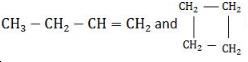QUESTION: 17

Which of the following is used in paints?

Solution:

It is a thermosetting plastic. It is obtained by condensation of ethylene glycol and phthalic acid or glycerol and phthalic acid. When its solution in a suitable solvent is evaporated, it leaves a tough but non-flexible film. It is, therefore, used in the manufacture of paints and lacquers.

QUESTION: 18

Which of the following statement is correct with respect to property of elements with an increase in atomic no. in the carbon family?

Solution:

In carbon family, C and Si show +4 oxidation state. The remaining elements of this group, i.e., Ge, Sn and Pb, however show two oxidation states +2 and +4 due to inert pair effect which arises due to ineffective shielding of the valence s-electrons by the intervening d- or f-electrons. Evidently, as the number of d and f-electrons increases down the group from Ge to Pb, the inert pair effect becomes more and more prominant. As a result, the stability of +4 oxidation state decreases while that of the +2 oxidation state increases from Ge to Pb.

QUESTION: 19

A non-volatile electrolyte dissolved in an aqueous solution in same molal proportion as non-electrolyte produces

Solution: The vant Hoff factor for the non electrolyte is equal to 1 whereas that of non volatile electrolyte is greater than 1. Hence all colligative properties will be increased.
QUESTION: 20

Which of the following alkali metals has the highest tendency for the half cell reaction?
M(g)   → M(g)+  + e-

Solution:

As the given reaction is oxidation reaction and lithium has highest oxidation potential, so it has highest tendency for this half reaction.

QUESTION: 21

Chloro ethane reacts with X to form diethyl ether. What is X

Solution:

Chloro Ethane Reacts with  Z  to form→  Di-Ethyl Ether

C₂H₅Cl   + C₂H₅ONa   →→  C₂H₅-O-C₂H₅  + NaCl

(Chloro-Ethane) + Sodium Ethoxide → Di-Ethyl Ether +Sodium Chloride

so, Z is Sodium Ethoxide

QUESTION: 22

Which among the following does not exhibit geometric isomerism

Solution:

Alkenes like 1-hexene when flipped from top to bottom they have identical structures and also they have C=CH2 unit which does not exist as cis- trans isomers.

QUESTION: 23

In colloidal state, particle size ranges from

Solution:
QUESTION: 24

Which is not amphoteric ?

Solution:

In chemistry, an amphoteric compound is a molecule or ion that can react both as an acid and as a base. Many metals (such as copper, zinc, chromium, tin, lead, aluminium, and beryllium) form amphoteric oxides or hydroxides.

QUESTION: 25

The IUPAC name of [Ni(NH3)4] [NiCl4] is

Solution:

As per the rules, the positive ions (cation) whether simple or complex, is named first followed by the negative ion (anion).

QUESTION: 26

Given: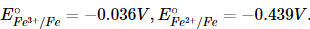. The value of standard electrode potential for the change,
Fe3+ (aq) + e→ Fe2+  (aq) will be

Solution: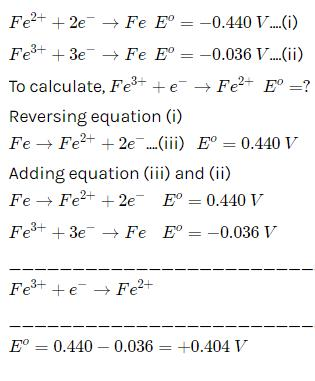QUESTION: 27

In the following reaction,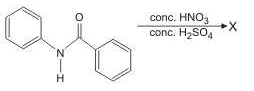the structure of the major product 'X' is

Solution: The mixture of the nitric acid and Sulphuric Acid gives No2+ which is an electrophile So it goes to electrophilic aromatic substitution in the compound which is more electropositive towards its ring in the option B nitrogen is giving its lone pair into the ring and increasing the electron density so to reduce the electron clouding known as steric inhibition or steric factor nucleophile attack on para position instead of Ortho which is very low yield
QUESTION: 28

Consider a titration of potassium dichromate solution with acidified Mohr’s salt solution using diphenylamine as indicator. The number of moles of Mohr’s salt required per mole of dichromate is

Solution: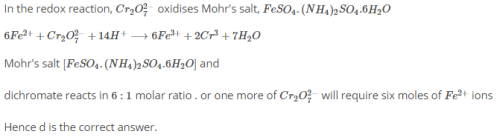QUESTION: 29

The reaction of P4 with X leads selectively to P4O6. The X is

Solution:
QUESTION: 30

The reagent(s) for the following conversion,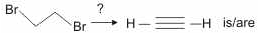Solution: